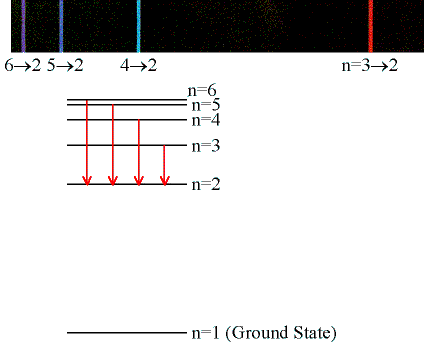# What wavelength of light will be emitted from a hydrogen atom for an electron that falls from n = 5 to n = 2 ?

Aug 19, 2016

$\textsf{\lambda = 434 \textcolor{w h i t e}{x} n m}$

#### Explanation:

We can use the Rydberg Expression:

$\textsf{\frac{1}{\lambda} = R \left[\frac{1}{n} _ {1}^{2} - \frac{1}{n} _ {2}^{2}\right]}$

$\textsf{\lambda}$ is the wavelength

$\textsf{R}$ is the Rydberg Constant which is $\textsf{1.097 \times {10}^{- 7} \textcolor{w h i t e}{x} {m}^{-} 1}$

$\textsf{{n}_{1}}$ is the lower energy level

$\textsf{{n}_{2}}$ is the higher energy level

Putting in the numbers:

$\textsf{\frac{1}{\lambda} = 1.097 \times {10}^{7} \left[\frac{1}{2} ^ 2 - \frac{1}{5} ^ 2\right]}$

$\textsf{\frac{1}{\lambda} = 1.097 \times {10}^{7} \left[0.25 - 0.04\right] = 0.2304 \times {10}^{7} \textcolor{w h i t e}{x} {m}^{-} 1}$

$\therefore$$\textsf{\lambda = 4.34 \times {10}^{- 7} \textcolor{w h i t e}{x} m}$

$\textsf{\lambda = 434 \textcolor{w h i t e}{x} n m}$

Transitions like this down to the n = 2 energy level form the "Balmer Series" of spectral lines.

These occur in the visible region of the electromagnetic spectrum.